•••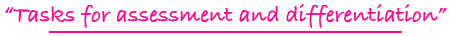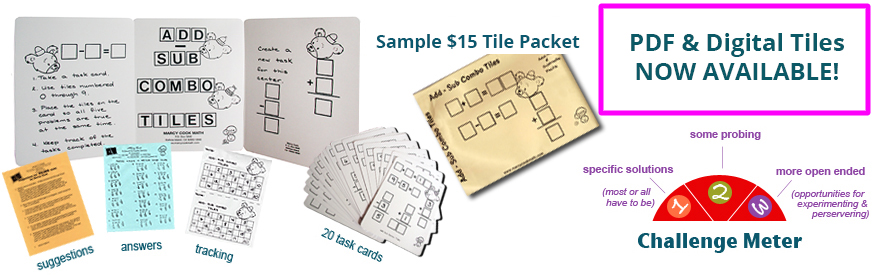Tiling Task Card Packets include 20 unique task cards on card stock, 3 backdrop cards, tracking sheets, suggestions and answer sheets.  These are contained in a printed manila envelope for convenient storing.  A set of number tiles 0 - 9 is  needed for each task card.

5 - 8

### FIND THE REMAINDER DIGITAL TILES

focusing on the remainder in division problems with a single-digit divisor

Challenge Level: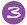DIGITAL FORMAT\$10.00

5 - 8

### FIND THE REMAINDER TILES

focusing on the remainder in division problems with a single-digit divisor

Challenge Level:PDF FORMAT
(You will receive both color and black and white files for printing.)\$10.00

K - 2

### FIRST COUNT, THEN ADD TILES

counting pictures and then performing the operation of addition by using symbols to represent numbers

Challenge Level: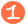\$15.00

K - 2

### FIRST COUNT, THEN ADD DIGITAL TILES

counting pictures and then performing the operation of addition by using symbols to represent numbers

Challenge Level:DIGITAL FORMAT\$10.00

K - 2

### FIRST COUNT, THEN ADD TILES

counting pictures and then performing the operation of addition by using symbols to represent numbers

Challenge Level:PDF FORMAT
(You will receive both color and black and white files for printing.)\$10.00

5 - 8

### FOUR WAY RELATIONSHIP TILES

completing if...then...relationship statements to find the cost of four items; enrichment problems requiring reasoning and perseverance

Challenge Level:\$15.00

4 - 7

adding common fractions with like and unlike denominators

Challenge Level: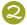\$15.00

4 - 7

adding common fractions with like and unlike denominators

Challenge Level:DIGITAL FORMAT\$10.00

4 - 7

adding common fractions with like and unlike denominators

Challenge Level:PDF FORMAT
(You will receive both color and black and white files for printing.)\$10.00

4 - 7

### FRACT-SUB TILES

subtracting common fractions with like and unlike denominators

Challenge Level:\$15.00

4 - 7

### FRACTION & NUMBER LINE DIGITAL TILES

comparing common fractions ( )using a visual model of a number line between zero and one

Challenge Level:DIGITAL FORMAT\$10.00

4 - 7

### FRACTION & NUMBER LINE TILES

comparing common fractions ( )using a visual model of a number line between zero and one

Challenge Level:PDF FORMAT
(You will receive both color and black and white files for printing.)\$10.00

4 - 7

### FRACTION COMPARISON TILES

comparing two fractions with different numerators and different denominators using visual models to record comparisons with symbols

Challenge Level:\$15.00

4 - 8

### FRACTION TILES

experimenting with number tiles to form common fractions; example: fractions equivalent to 3/4

Challenge Level:\$15.00

4 - 8

### FRACTIONAL PART TILES

finding fractional parts of whole numbers; examples: 1/3 of 39; less than 1/4 of 100

Challenge Level:\$15.00

3 - 6

### FUNCTION TILES I

figuring and applying rules for functions by looking at input and output numbers: addition, subtraction, multiplication, & division

Challenge Level:\$15.00

5 - 8

### FUNCTION TILES II

figuring and applying rules for functions by looking at input and output numbers: functions include 2 operations such as x2 + 1, squaring, square roots

Challenge Level:\$15.00

5 - 8

### F(X) TILES

finding x and y in T table fashion when given a function of x, such as f(x) = 3x

Challenge Level:\$15.00

1 - 3

### GRAPH TILES

forming addition and subtraction problems from data gathered from reading a picture graph

Challenge Level:\$15.00

1 - 3

### GREATER, EQUAL, LESS THAN DIGITAL TILES

comparing two numbers using the greater than and less than symbols as well as the equal sign

Challenge Level:DIGITAL FORMAT